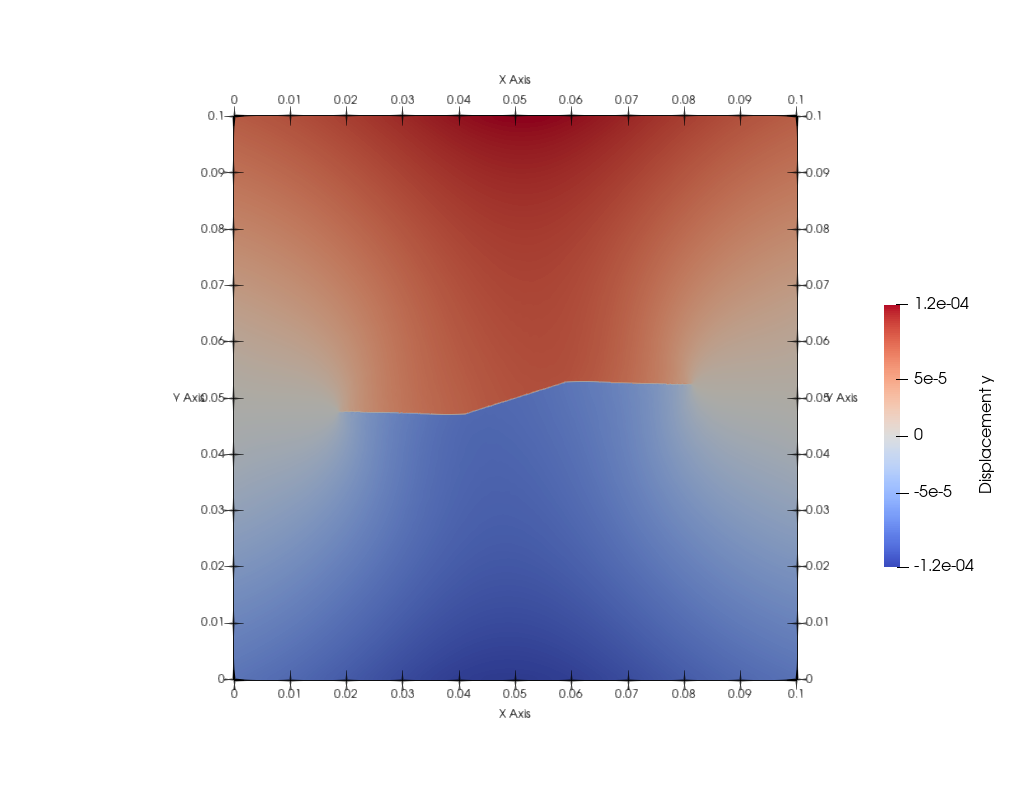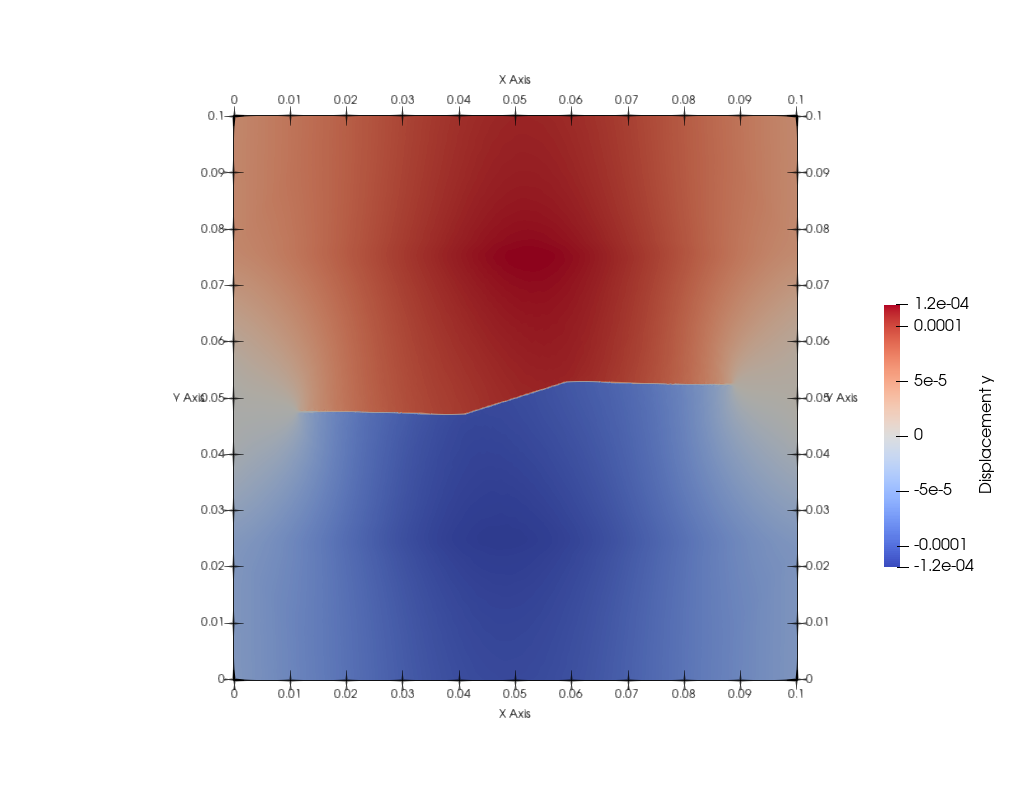Sometimes for dynamic simulations it is interesting to see how the simulation behaves after the final load was applied. Therefore, the parameter Relaxation_Steps is provided. This parameter defines the number of the time steps to be executed after the final load is applied. In this case the defined load will be successive applied over 50000 time steps until the final time  Final_Time: 0.001000 is reached. After the the load drops to zero and the simulations proceeds for 501 time steps using the the same time step size.

Model:
Dimension: 2
Discretization_Type:
Spatial: finite_difference
Time: velocity_verlet
Final_Time: 0.001000
Time_Steps: 50000
Relaxation_Steps: 501


### Results

This is the plot of the displacement in y-direction at the final time Final_Time: 0.001000:This is the plot of the displacement in y-direction after the 501 relaxation steps:Since the crack develops further, but the maximal displacement is the same we see interal forces after applying the load. This shows that the simualtion has still dynamic effects and the time step size should be reduced to do not see this effects.